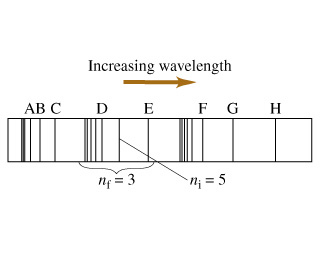# Problem: The spectrum of a hypothetical atom is shown in the figure. Three distinct spectral series are shown, with the center series corresponding to transitions to a final state of nf = 3. The indicated spectral line corresponds to the transition from an initial state of ni = 5 to the final state nf = 3.QUESTION:1) Which of the lettered spectral lines corresponds to the transition from ni = 6 to nf = 3?A, B, C, D E, F, G, H2) Which of the lettered spectral lines corresponds to the transition from ni = 5 to nf = 4?A, B, C, D, E, F, G, H3) Which of the lettered spectral lines corresponds to the transition from ni = 5 to nf = 2?A, B, C, D, E, F, G, H

###### FREE Expert Solution

1)

The spectral lines produced in the transition from ni = 6 to nf = 3 is of more energy compared to a transition from ni = 5 to nf = 3.

The wavelength for the first transition is also less than the wavelength for the second transition.

Therefore, ni = 6 to nf = 3 has a decreasing wavelength.###### Problem Details

The spectrum of a hypothetical atom is shown in the figure. Three distinct spectral series are shown, with the center series corresponding to transitions to a final state of nf = 3. The indicated spectral line corresponds to the transition from an initial state of ni = 5 to the final state nf = 3.

QUESTION:1) Which of the lettered spectral lines corresponds to the transition from ni = 6 to nf = 3?

A, B, C, D E, F, G, H

2) Which of the lettered spectral lines corresponds to the transition from ni = 5 to nf = 4?

A, B, C, D, E, F, G, H

3) Which of the lettered spectral lines corresponds to the transition from ni = 5 to nf = 2?
A, B, C, D, E, F, G, H